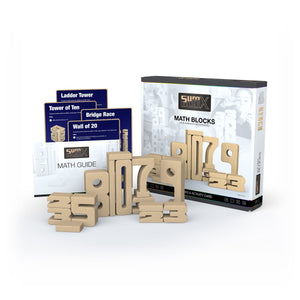Free shipping for orders over £50 use code SPRING at checkout# SumBlox Home Set

Regular price £119.99
Unit price  per
Shipping calculated at checkout.

43 Blocks + Activity Card

43 hardwood blocks with a folded activity card that kids can follow.

### For what age are SumBlox best suited?

SumBlox are great for children and adults of all ages but are best suited, academically, for children ages 2-12.

### What mathematical concepts can SumBlox teach?

Students can learn a wealth of information through play and hands-on exploration. These topics include:

##### Number Sense
• Number recognition
• One-to-one correspondence
• Compare numbers
• Subitizing
• Counting
• Cardinality
• Number sequence
• Hierarchical Inclusion
• Understanding our base-ten system
• Place value
• Measuring Lengths
• Reason abstractly and quantitatively
• Understand Equivalence
• Directly compare two objects with a measurable attribute in common
• Algebraic Thinking
• Describe measurable attributes of objects, such as length or weight
• Classify objects into given categories
##### Addition / Subtraction
• Solve Addition and Subtraction word problems using objects
• Compose and decompose numbers
• Find missing addends
• Skip counting
##### Multiplication / Division
• Define multiplication; emphasis on multiples
• Find factors of whole numbers and use commutative property of multiplication
• Classify whole numbers (greater than one) as prime or composite
• Draw conclusions about the factors of a whole number
• Use associative property of multiplication
• Use distributive property of multiplication
##### Fractions / Adding Fractions
• Define denominator of fractions
• Define numerator of fractions within halves, fourths, and eights
• Find equivalent fractions within thirds, sixths, ninths, and twelfths
• Compare two fractions using inequality symbols
• Compare and order three fractions using compound inequalities
• Find the value of a fraction of a whole number
• Find the fraction, or part, a given value is of a whole value
• Add two fractions with the same denominator to get a sum up to one whole and fully decompose a given fraction
• Add two fraction addends with the same denominator to get a sum greater than one whole
• Add multiple fraction addends to find sums greater than one whole and represent sums in improper form and mixed numbers
• Add two fraction addends with different denominators (only having to scale one of the addends)
• Add three fraction addends with different denominators (only having to scale two of the addends)
• Add two fractions addends with different denominators (having to scale all addends to find a common denominator)